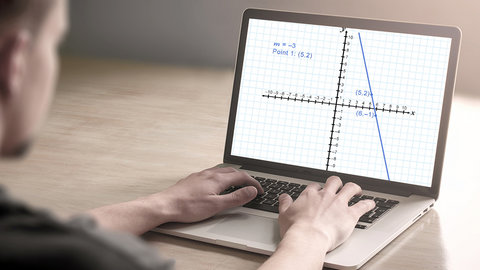Graphing Linear Equations, Part 1 Episode 10 of Algebra I

The Great CoursesRelated videos

Graphing Linear Equations, Part 2
Episode 11 of Algebra I
A more versatile approach to writing the equation of a line is the point-slope form, in which only two points are required, and neither needs to intercept the y axis. Work through several examples and become comfortable determining the equation using the line and the line using the equation.
Solving Linear Equations, Part 1
Episode 7 of Algebra I
In this lesson, work through simple one- and two-step linear equations, learning how to isolate the variable by different operations. Professor Sellers also presents a word problem involving a two-step equation and gives tips for how to solve it.
Solving Linear Equations, Part 2
Episode 8 of Algebra I
Investigating more complicated examples of linear equations, learn that linear equations fall into three categories. First, the equation might have exactly one solution. Second, it might have no solutions at all. Third, it might be an identity, which means every number is a solution.
Systems of Linear Equations, Part 1
Episode 15 of Algebra I
When two lines intersect, they form a system of linear equations. Discover two methods for finding a solution to such a system: by graphing and by substitution. Then try out a real-world example, involving a farmer who wants to plant different crops in different proportions.
Systems of Linear Equations, Part 2
Episode 16 of Algebra I
Expand your tools for solving systems of linear equations by exploring the method of solving by elimination. This technique allows you to eliminate one variable by performing addition, subtraction, or multiplication on both sides of an equation, allowing a straightforward solution for the remaining variable.
Solving Word Problems with Linear Equations
Episode 13 of Algebra I
Linear equations reflect the behavior of real-life phenomena. Practice evaluating tables of numbers to determine if they can be represented as linear equations. Conclude with an example about the yearly growth of a tree. Does it increase in size at a linear rate?
Linear Equations for Real-World Data
Episode 14 of Algebra I
Investigating more real-world applications of linear equations, derive the formula for converting degrees Celsius to Fahrenheit; determine the boiling point of water in Denver, Colorado; and calculate the speed of a rising balloon and the time for an elevator to descend to the ground floor.
Graphing Rational Functions, Part 1
Episode 30 of Algebra I
Examine the distinctive graphs formed by rational functions, which may form vertical or horizontal curves that aren't even connected on a graph. Learn to identify the intercepts and the vertical and horizontal asymptotes of these fascinating curves.
Graphing Rational Functions, Part 2
Episode 31 of Algebra I
Sketch the graphs of several rational functions by first calculating the vertical and horizontal asymptotes, the x and y intercepts, and then plotting several points in the function. In the final exercise, you must simplify the expression in order to extract the needed information.
Principles of Graphing in 2 Dimensions
Episode 6 of Algebra I
Using graph paper and pencil, begin your exploration of the coordinate plane, also known as the Cartesian plane. Learn how to plot points in the four quadrants of the plane, how to choose a scale for labeling the x and y axes, and how to graph a linear equation.